CBSE Class 12 Sample Paper for 2021 Boards

Class 12
Solutions of Sample Papers and Past Year Papers - for Class 12 Boards

## −2𝑦 + 𝑧 = 7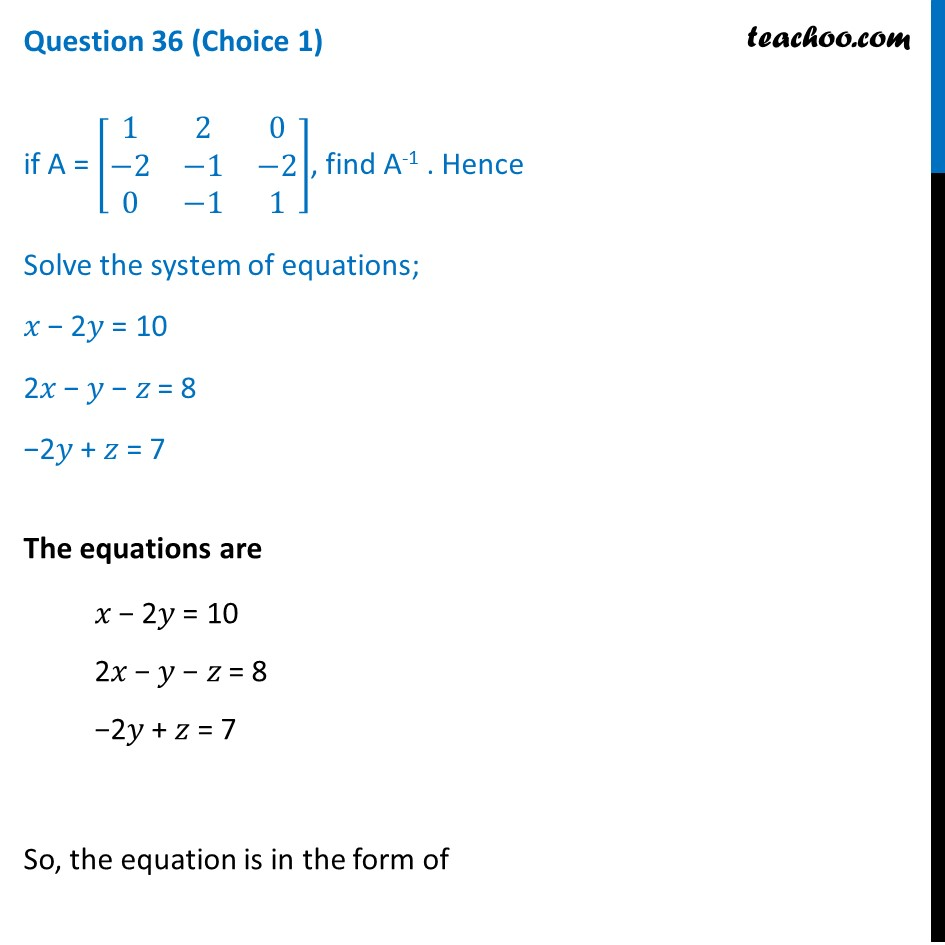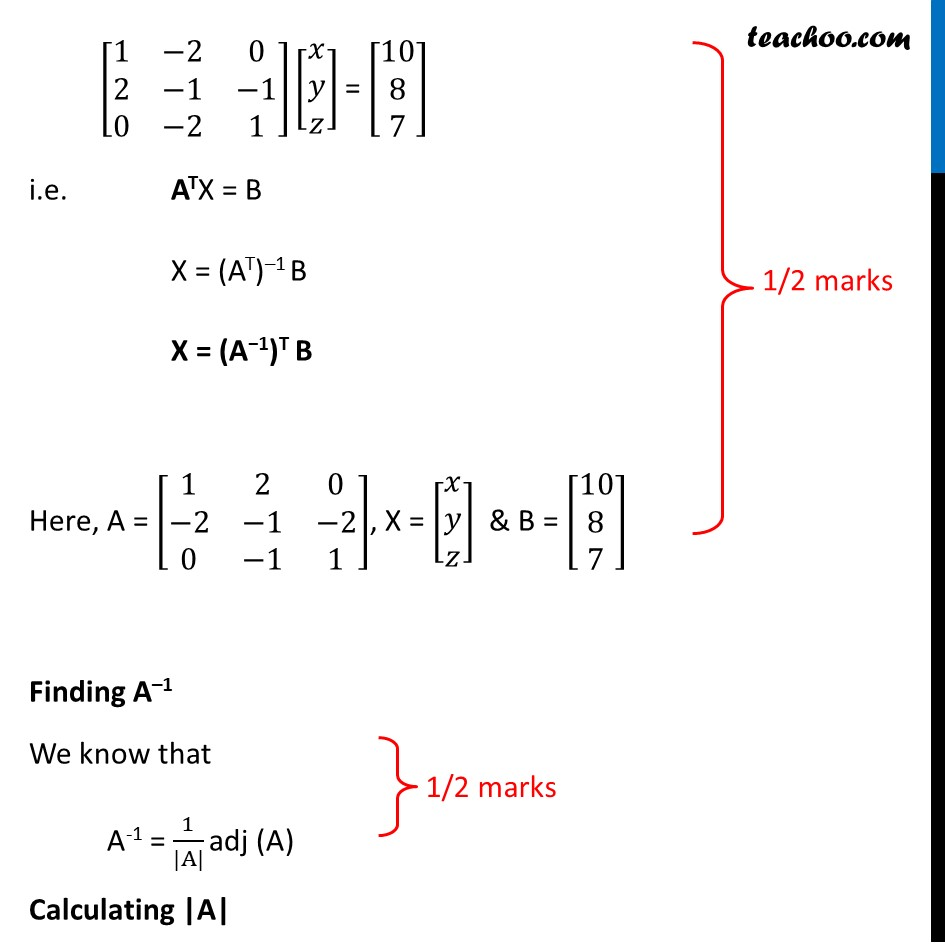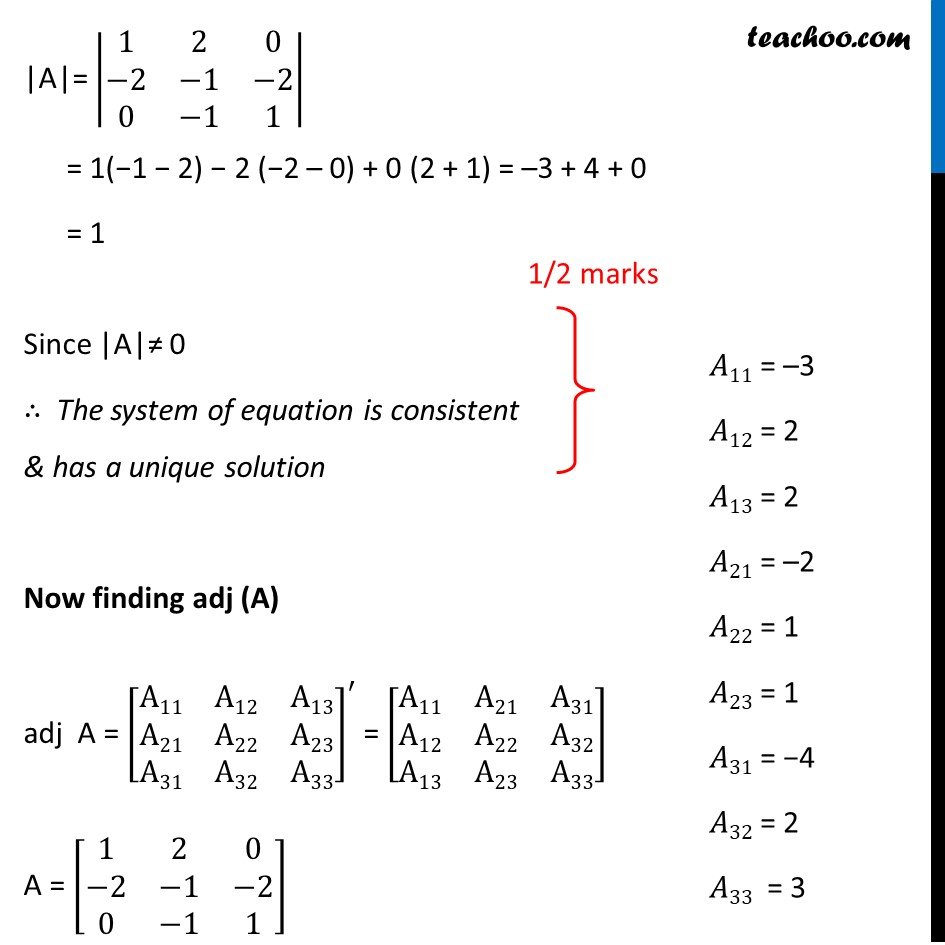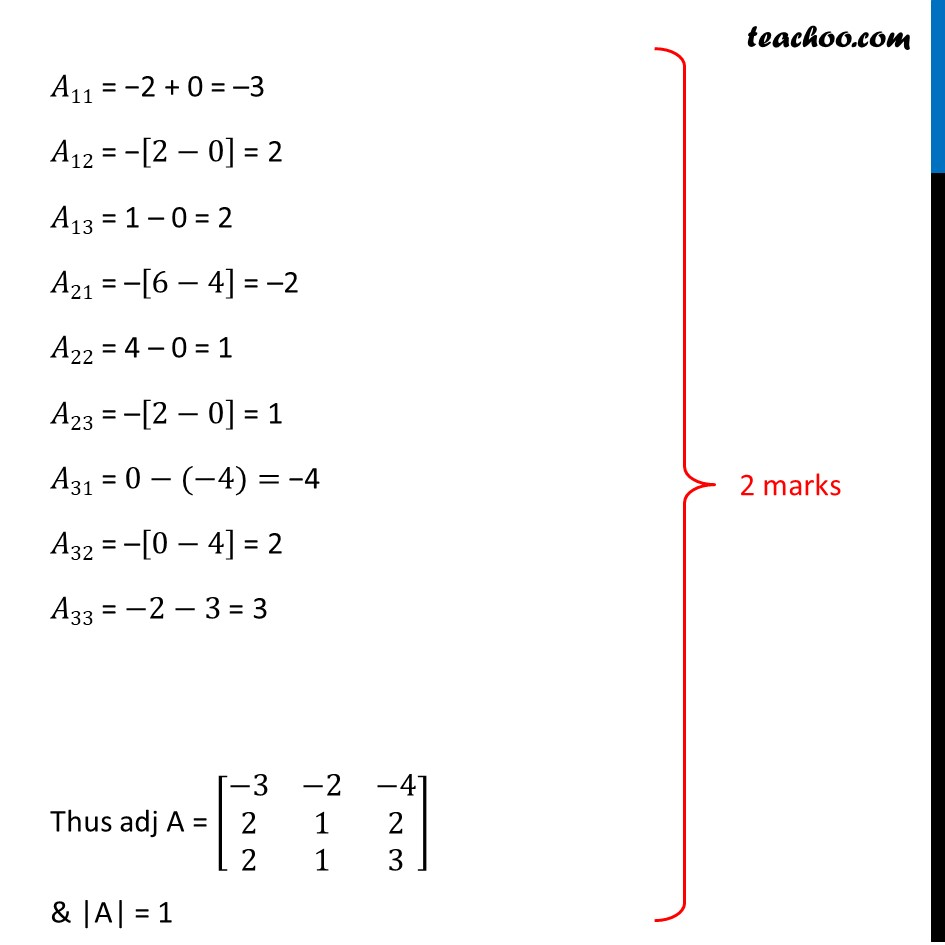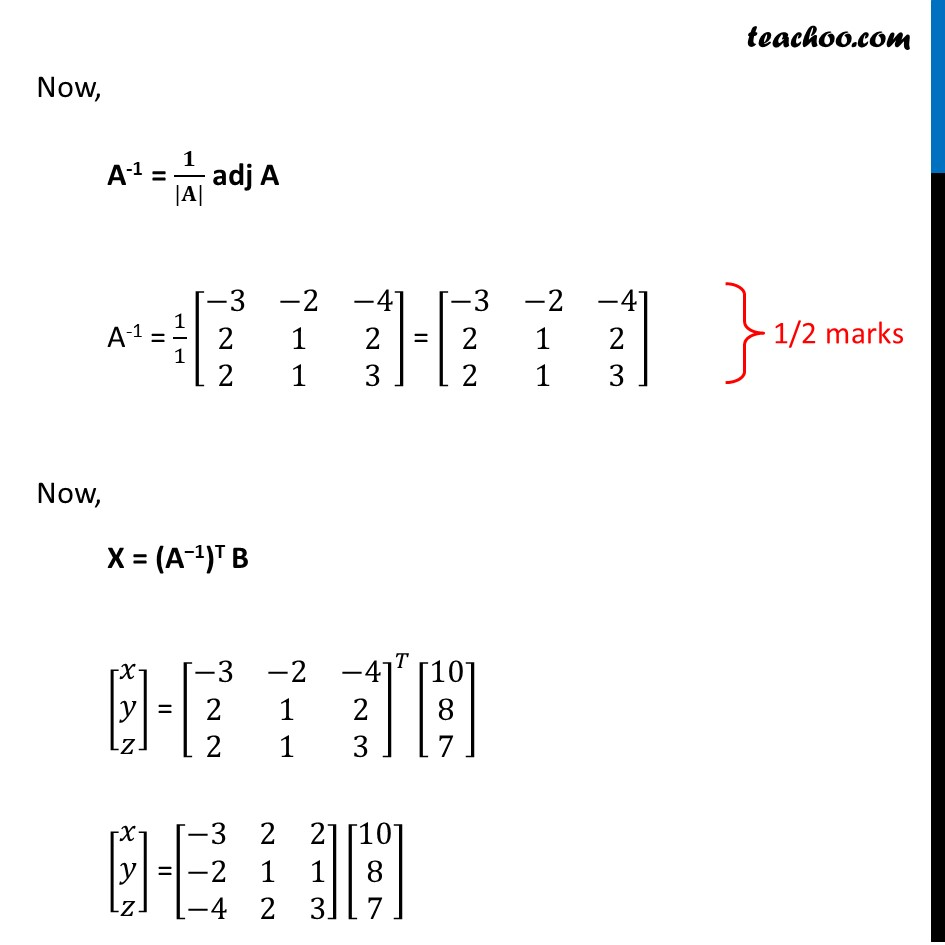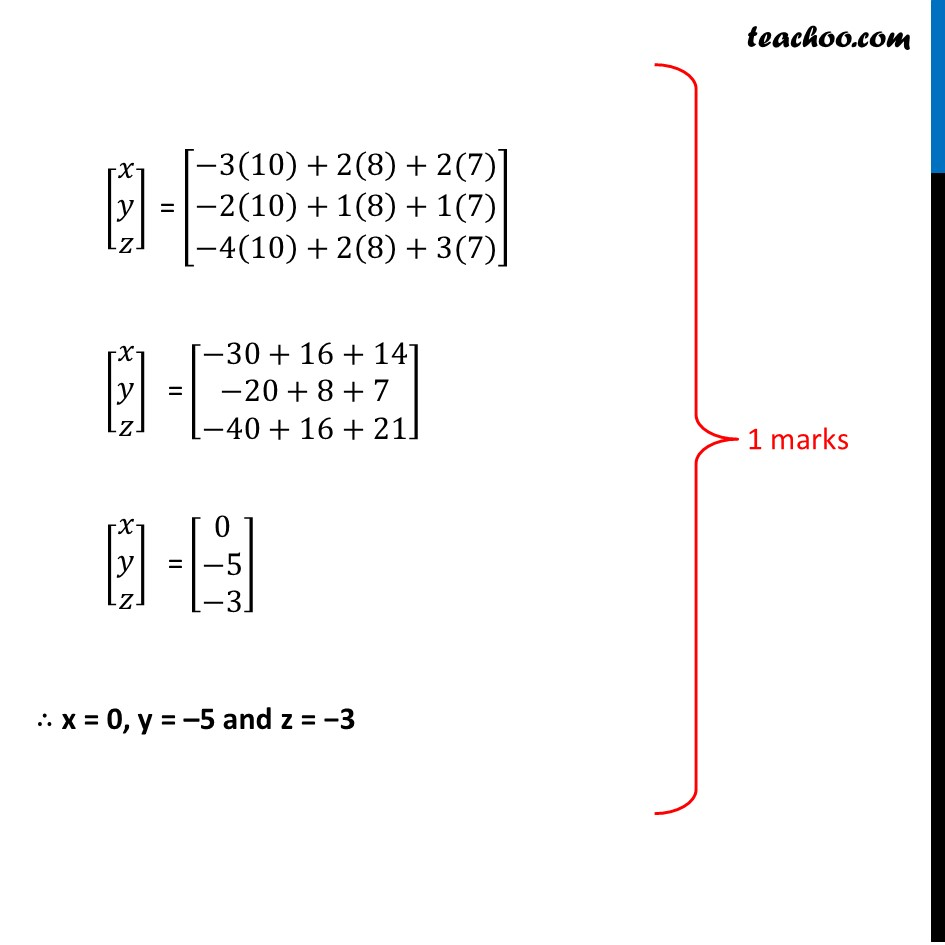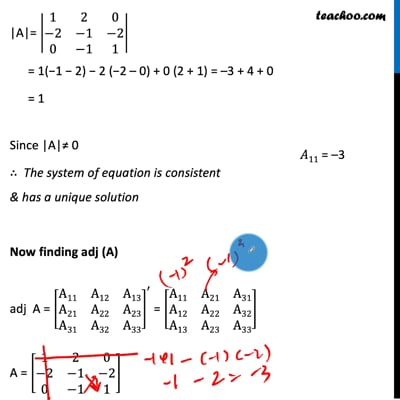This video is only available for Teachoo black users

Note : This is similar to Question 33 of CBSE Sample Paper Maths Class 12 - 2020

Introducing your new favourite teacher - Teachoo Black, at only ₹83 per month

### Transcript

Question 36 (Choice 1) if A = [■8(1&2&0@−2&−1&−2@0&−1&1)], find A-1 . Hence Solve the system of equations; 𝑥 − 2𝑦 = 10 2𝑥 − 𝑦 − 𝑧 = 8 −2𝑦 + 𝑧 = 7 The equations are 𝑥 − 2𝑦 = 10 2𝑥 − 𝑦 − 𝑧 = 8 −2𝑦 + 𝑧 = 7 So, the equation is in the form of [■8(1&−2&0@2&−1&−1@0&−2&1)][■8(𝑥@𝑦@𝑧)] = [■8(10@8@7)] i.e. ATX = B X = (AT)–1 B X = (A−1)T B Here, A = [■8(1&2&0@−2&−1&−2@0&−1&1)], X = [■8(𝑥@𝑦@𝑧)] & B = [■8(10@8@7)] Finding A–1 We know that A-1 = 1/(|A|) adj (A) Calculating |A| |A|= |■8(1&2&0@−2&−1&−2@0&−1&1)| = 1(−1 − 2) − 2 (−2 – 0) + 0 (2 + 1) = –3 + 4 + 0 = 1 Since |A|≠ 0 ∴ The system of equation is consistent & has a unique solution Now finding adj (A) adj A = [■8(A11&A12&A13@A21&A22&A23@A31&A32&A33)]^′ = [■8(A11&A21&A31@A12&A22&A32@A13&A23&A33)] A = [■8(1&2&0@−2&−1&−2@0&−1&1)] 𝐴11 = −2 + 0 = –3 𝐴12 = −[2−0] = 2 𝐴13 = 1 – 0 = 2 𝐴21 = –[6−4] = –2 𝐴22 = 4 – 0 = 1 𝐴23 = –[2−0] = 1 𝐴31 = 0−(−4)= −4 𝐴32 = –[0−4] = 2 𝐴33 = −2−3 = 3 Thus adj A = [■8(−3&−2&−4@2&1&2@2&1&3)] & |A| = 1 Now, A-1 = 𝟏/(|𝐀|) adj A A-1 = 1/1 [■8(−3&−2&−4@2&1&2@2&1&3)] = [■8(−3&−2&−4@2&1&2@2&1&3)] Now, X = (A−1)T B [■8(𝑥@𝑦@𝑧)] = [■8(−3&−2&−4@2&1&2@2&1&3)]^𝑇 [■8(10@8@7)] [■8(𝑥@𝑦@𝑧)] =[■8(−3&2&2@−2&1&1@−4&2&3)][■8(10@8@7)] [■8(𝑥@𝑦@𝑧)]" =" [█(−3(10)+2(8)+2(7)@−2(10)+1(8)+1(7)@−4(10)+2(8)+3(7))] " " [■8(𝑥@𝑦@𝑧)]" =" [■8(−30+16+14@−20+8+7@−40+16+21)] " " [■8(𝑥@𝑦@𝑧)]" =" [■8(0@−5@−3)] "∴ x = 0, y = –5 and z = −3"